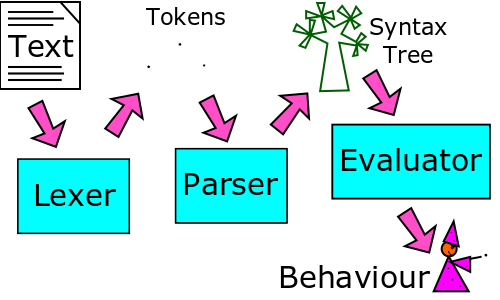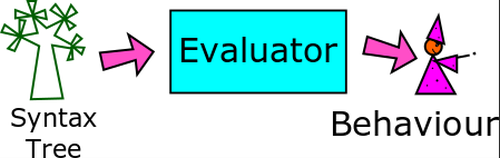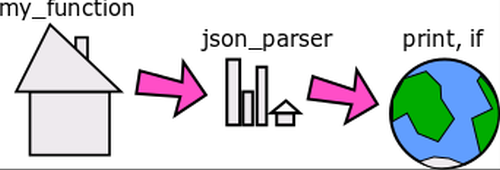# How to Write a Programming Language: Part 3, The Evaluator

How to Write a Programming Language: Part 3, The Evaluator

By Andy Balaam

We’ve parsed our tokens: now we need turn them into values. Andy Balaam continues writing a programming language with the evaluator.

This is the third part of our series on writing a programming language. In part one we broke up the code into chunks like numbers, strings and symbols (lexing), and in part two we assembled those parts into a tree structure (parsing). Now we are ready to start understanding and evaluating the parts of that tree structure to produce values and behaviour.By the end of this article you will have seen all the most important parts of a programming language, and be ready to write your own!

## Recap – lexing and parsing

The lexer and parser take some text like this:

`  print( x + 2 );`

and break it into parts, and then assemble it into a tree structure like this:

```  ("call",
("symbol", "print"),
[
("operation",
"+",
("symbol", "x"),
("number", "2")
)
]
)```

Cell is written in Python and uses Python tuples to hold all the structures it represents. Each tuple contains a string representing its type as the first element, and then other information in the other elements of the tuple.

So far, we have seen tuples representing tokens coming out of the lexer, and tuples representing syntax trees coming out of the parser. This time we will see more tuples, representing values that have been worked out by the evaluator.

## The evaluator calculates valuesThe evaluator starts at the leaves of the syntax tree and calculates the values of the leaves, then combines together leaves and branches until it ends up with a single value. On the way it may have produced some side effects such as printing something out.## Scope

Before we look at how the evaluator works out values, we must look at the idea of scope. Scope describes what names can be seen where in our code. Cell, like almost all modern programming languages, uses ‘lexical’ scope, which means the values you can see are dictated by the position of the code in the text on the screen.

So, for example, this snippet of Cell code:

```  x = "World!";
myfn = {
x = "Hello,";
print( x );
};
myfn();
print( x );```

prints:

```  Hello,
World```

because the value of ``` x ``` inside the function ``` myfn ``` is set to ``` "Hello," ``` within the function definition, but it reverts to ``` "World!" ``` in code that is outside that block.

This more complicated example:

```  outerfn = {
x = 12;
innerfn = {
print(x);
};
innerfn;
};

thing = outerfn();
thing();```

prints ``` "12" ``` because the function ``` innerfn ``` carries the values it knows about with it, meaning that when we call the function returned by ``` outerfn() ``` , which is actually ``` innerfn ``` because that is what is returned by ``` outerfn ``` when we call it, it runs the ``` print(x) ``` line and it still knows what ``` x ``` is. Functions that are carrying their values with them are called Closures, and the set of values that is passed around is called an Environment. Environments are key to the way the evaluator works.

## Environments

An environment is a namespace that holds all the symbols that are defined in your program. As illustrated here, each piece of code operates inside a local environment (such as the current function) but can also access symbols from outer environments (such as an outer function) and the global environment, that contains important symbols such as the ``` if ``` function.This structure is provided in Cell by a class called ``` Env ``` . It takes a parent environment as a constructor argument, which it holds in ``` self.parent ``` . To look up a name we call the ``` get() ``` method:

```  class Env:
# ...
def get(self, name):
if name in self.items:
return self.items[name]
elif self.parent is not None:
return self.parent.get(name)
else:
return None```

This method checks whether a symbol is defined locally, and if not, it asks the parent environment. It gives up when it gets to the global environment, which has ``` None ``` for its ``` self.parent ``` value.

Defining a symbol means calling ``` set() ``` , which is simpler:

```  class Env:
# ...
def set(self, name, value):
self.items[name] = value```

So newly defined symbols are always defined in the local environment, and don’t leak out into wider scopes.

## The evaluator

Listing 1 shows the main logic of the evaluator. You can see the full code at https://github.com/andybalaam/cell/blob/master/pycell/eval_.py , but here we can see the main structure is very similar to the code we saw in the previous two parts – a large ``` if ``` - ``` elif ``` block responding differently to the various possible structures.

 ```def eval_expr(expr, env): typ = expr if typ == "number": return ("number", float(expr)) elif typ == "string": return ("string", expr) elif typ == "none": return ("none",) elif typ == "operation": return _operation(expr, env) elif typ == "symbol": name = expr ret = env.get(name) if ret is None: raise Exception( "Unknown symbol '%s'." % name) else: return ret elif typ == "assignment": var_name = expr val = eval_expr(expr, env) env.set(var_name, val) return val elif typ == "call": return _function_call(expr, env) elif typ == "function": return ("function", expr, expr, Env(env)) else: raise Exception( "Unknown expression type: " + str(expr)) ``` Listing 1

In the evaluator, the ``` eval_expr() ``` function takes in an expression to evaluate, and the environment in which to work. Without an environment we can’t do anything since we don’t know where to look up the symbols that are being used in the code. The environment is an instance of the ``` Env ``` class we saw earlier, and the expression is a Python tuple representing part of a syntax tree.

The first part of the tuple tells us the type of syntax tree section we have. We place this into a variable called ``` typ ``` , and use it in the ``` if ``` block.

## Ordinary values

If we are evaluating a number, we use Python’s ``` float() ``` function to convert the string form that was captured in the lexer token (as ``` expr ``` , the second value in the tuple) into a Python number. This means we can do arithmetic with it later if we need to, and illustrates the fact that the evaluator is where the textual and structural forms of the code are converted into ‘meaning’ such as finding actual numbers and looking up the values of symbols.

If we find a string, we have very little to do, since the value of a string looks identical to its form as a lexer token – i.e. it is a tuple of two values, the first of which is ``` "string" ``` and the second is the contents of that string.

Next we deal with a special case – there is a special type of value in Cell that is called ``` None ``` – we inject this value into the global environment with the name ``` None ``` , and the Python tuple to represent its value is ``` ("none",) ``` i.e. a tuple with just one value in it to represent a special none type. In Cell, ``` None ``` is used to describe a missing or empty value. If we find a ``` None ``` value like this, we simply return a similar ``` None ``` value from ``` eval_expr ``` .

The next type of syntax tree we handle is ``` "operation" ``` – this represents an arithmetic operation like ``` "+" ``` or ``` "*" ``` . We call a dedicated function ``` _operation() ``` to deal with this, which is shown in listing 2.

 ```def _operation(expr, env): arg1 = eval_expr(expr, env) arg2 = eval_expr(expr, env) if expr == "+": return ("number", arg1 + arg2) elif expr == "-": return ("number", arg1 - arg2) elif expr == "*": return ("number", arg1 * arg2) elif expr == "/": return ("number", arg1 / arg2) else: raise Exception( "Unknown operation: " + expr) ``` Listing 2

The ``` _operation() ``` function takes in an expression and environment just like ``` eval_expr ``` , and it looks at ``` expr ``` to find out what kind of operation is being asked for. As we saw in the previous article, this was populated by the parser and can be ``` "+" ``` , ``` "-" ``` , ``` "*" ``` or ``` "/" ``` . In each case, we evaluate the expressions on the left and right of the operator and place them into variables ``` arg1 ``` and ``` arg2 ``` , and then combine them together using the appropriate Python arithmetic operator. If the rules of arithmetic in Cell were different from those in Python, this is where we would see the difference. Similarly, if we chose to use some other class to represent numbers, we would have seen that being used when we found a ``` "number" ``` type, instead of the ``` float() ``` function. In fact, because Cell is designed to be simple to implement, we choose to use Python’s float type and built-in arithmetic for all the numeric operations.

Back in the main code (listing 1) we see the next type is ``` "symbol" ``` . This means we found a symbol like ``` my_function ``` in the code, or a built-in symbol like ``` print ``` or ``` if ``` . To evaluate it, we simply look it up in the environment using ``` Env ``` ’s ``` get() ``` method that we saw earlier. If we can’t find it, we throw an exception, producing a crude error message for the user.

Similarly, if the type is ``` "assignment" ``` , we have found code like ``` "x = 3" ``` , and we use the environment’s ``` set() ``` method to store the value inside the symbol we were given. We make sure to evaluate the value before storing it, by calling ``` eval_expr() ``` again. In this case, and in other cases, we call ``` eval_expr ``` from inside ``` eval_expr ``` . This is called recursion, and makes perfect sense if you don’t think about it too much, or, alternatively, if you think about it a lot.

## Functions

The last two types to deal with both concern functions. First, ``` "call" ``` means we are looking at a function call, something like ``` "my_fn(3)" ``` . We deal with this in a separate function called ``` _function_call ``` , shown in listing 3.

 ```def _function_call(expr, env): fn = eval_expr(expr, env) args = list((eval_expr(a, env) for a in expr)) if fn == "function": params = fn fail_if_wrong_number_of_args(expr, params, args) body = fn fn_env = fn new_env = Env(fn_env) for p, a in zip(params, args): new_env.set(p, a) return eval_list(body, new_env) elif fn == "native": py_fn = fn params = inspect.getargspec(py_fn).args fail_if_wrong_number_of_args(expr, params[1:], args) return fn(env, *args) else: raise Exception( "Attempted to call something that is not a function: %s" % str(fn) ) ``` Listing 3

The ``` _function_call ``` function (did I mention that writing a programming language can get confusing when you start writing functions about functions, or variables containing variables?) evaluates the function object that is being called and checks its type.

The type will be either ``` "function" ``` or ``` "native" ``` . The ``` "function" ``` type means that this is a normal function written in Cell. In order to run it, we check we have been given the right number of arguments, and then create a temporary environment based on the environment carried around by the function itself (recall the discussion of scope and closures earlier). Next it puts the argument values into that environment using the names provided in the function definition, and then calls ``` eval_list ``` . It is not shown here, but ``` eval_list ``` just evaluates each line of the function one by one. It actually ignores the values of all those lines except the last one, which it uses as the function’s return value.

A ``` "native" ``` type is a function that is not written in Cell, but instead is provided as part of Cell’s implementation. This means the function is written in Python (because Cell is written in Python). In this case, ``` fn ``` is a Python function. We check the number of arguments again, and call the function, passing in the environment and the arguments. Python functions that provide native Cell functions actually take one more argument in Python than you see in Cell, because the first argument is the environment in which to run. We don’t create a sub-environment in which to run in this case, because native functions can do all kinds of magic, like modifying the environment in which they are running. Writing these functions is slightly odd because the arguments passed in are tuples representing Cell values, rather than simple Python types, and any symbols etc. need to be looked up in the environment provided.

Back in listing 1, the last type we deal with is ``` "function" ``` . So far we’ve only dealt with calling functions, but this is about the definition of a function – in Cell that means code inside curly braces. In fact, most of the hard work of defining the function has been done by the parser, which made us a list of argument names and expressions that make up the body of the function. All we need to do in the evaluator is wrap all that up with a new ``` Env ``` object that is the environment passed around with the function. This means if we return a function definition from another function, it can still access the variables it could see when it was defined because its environment (and the parent environments) are held with it. We rely on Python’s object references to make sure the values we are interested in are still available when we use them.

The ``` else ``` part of listing 1 throws an exception because we have found a syntax tree that we don’t recognise (in the famous last words of all programmers, this should never happen) and we are done.

## Side effects

With all this discussion of finding values, it seems strange to say that most programming languages, including Cell, actually do nothing with the values they find. In order to make a useful program, the programmer must use the values to produce ‘side effects’ – things that make something happen in the world outside the program. In Cell, we have a native function called ``` "print" ``` that prints out values we have calculated. Most other languages have lots of available side effects such as creating and modifying files, displaying windows, and making sounds.

## Summary

We’ve completed our journey: this time we saw how to take a syntax tree and turn it into meaningful values. When we combine this with being able to break code into separate chunks (lexing) and building those chunks into a syntax tree (parsing), we’ve covered all the basic building blocks needed to write an interpreter. Are you ready to design your own language?

You can find all the code for Cell at https://github.com/andybalaam/cell , and I would to hear from you if you have made your own language – let me know through GitHub or Twitter on @andybalaam or in the fediverse on andybalaam@mastodon.social, and check out a video series about Cell on my YouTube page at https:// www.youtube.com/user/ajbalaam.

Andy is happy as long as he has a programming language and a problem. He finds over time he has more and more of each. You can find his open source projects at artificialworlds.net